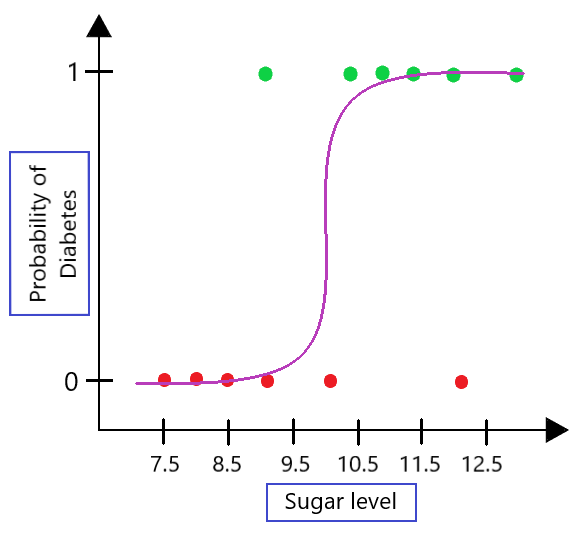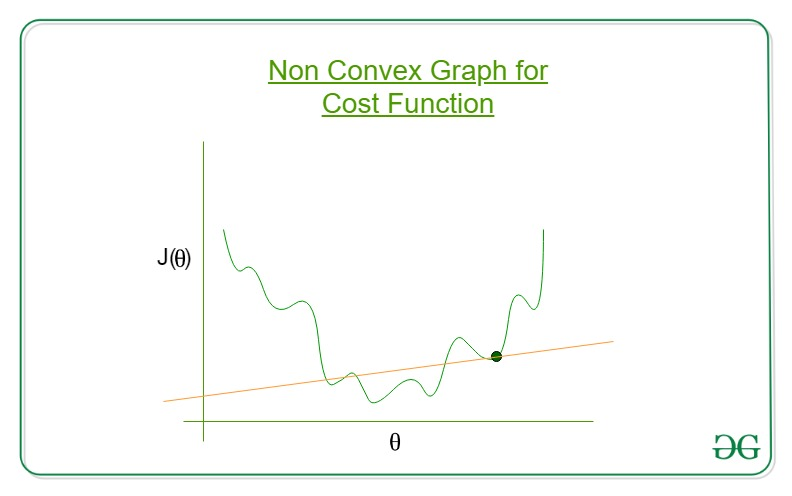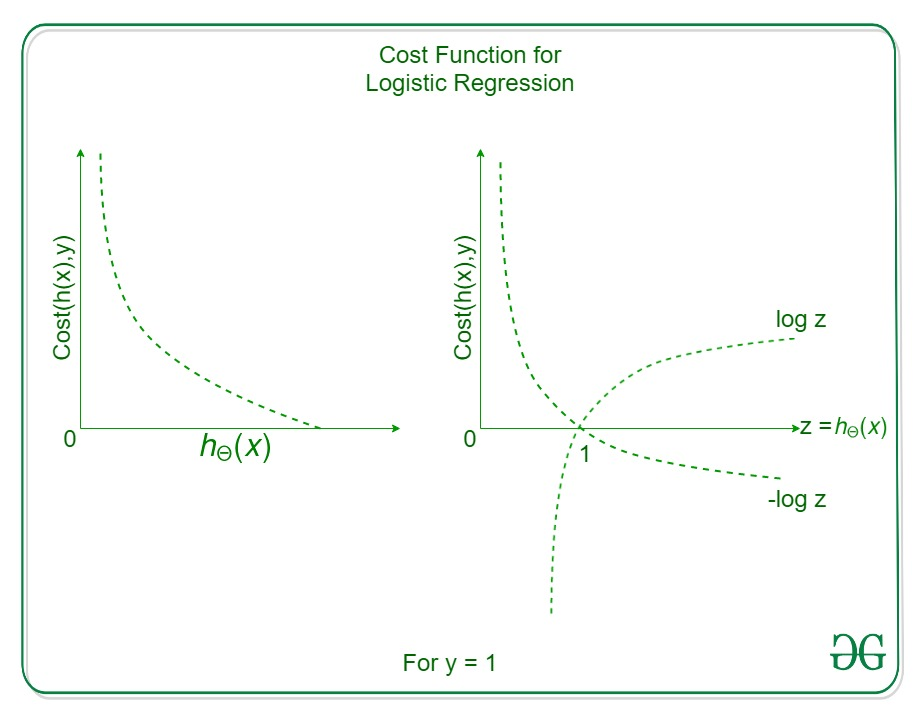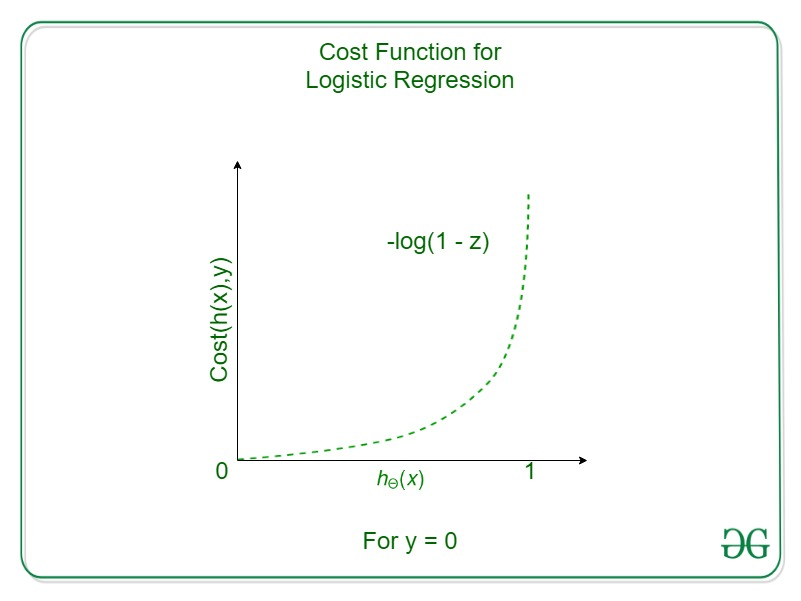# Cost function in Logistic Regression in Machine Learning

Logistic Regression is one of the simplest classification algorithms which we learn while exploring machine learning algorithms. But we use cross entropy instead of the mean squared error. In this article, we will explore the main reason behind it.

## Why do we need Logistic Regression?

Even when we have a linear regression algorithm then why do we need another algorithm that is logistic regression? To answer this question first we need to understand the problem behind the linear regression for the classification task.## This image need changes

From the above graph, we can observe that the linear regression line is not a good fit as compared to the graph of the sigmoid function. Also if we try to explore the cost function graph on which we try to optimize the cost function is a non-convex graph.Weights getting stuck at local minima instead of the Global Minima

While dealing with an optimization problem with such a graph at hand then we face the problem of getting stuck at the local minima instead of the global minima. Before moving forward let’s understand the two most important terms which are very important in the case of logistic regression.

### Sigmoid Function

We can also view this as a non-linear transformation of the linear regression line. By using this we get the values confined between the range 0 and 1. Also, our target class is also 0 and 1 so, the values which we get are between this range, and by applying some thresholding(if the predicted value is greater than 0.5 then predict 1 else 0) we can set the predicted values to either 0 or 1.### Log Loss or Cross Entropy Function

Log loss is a classification evaluation metric that is used to compare different models which we build during the process of model development. It is considered one of the efficient metrics for evaluation purposes while dealing with the soft probabilities predicted by the model.## Cost function for Logistic Regression

In the case of Linear Regression, the Cost function is:But for Logistic Regression,It will result in a non-convex cost function as shown above. So, for Logistic Regression the cost function we use is also known as the cross entropy or the log loss.Case 1: If y = 1, that is the true label of the class is 1. Cost = 0 if the predicted value of the label is 1 as well. But as hθ(x) deviates from 1 and approaches 0 cost function increases exponentially and tends to infinity which can be appreciated from the below graph as well.Cost Function for Logistic Regression for the case y=1

Case 2: If y = 0, that is the true label of the class is 0. Cost = 0 if the predicted value of the label is 0 as well. But as hθ(x) deviates from 0 and approaches 1 cost function increases exponentially and tends to infinity which can be appreciated from the below graph as well.Cost Function for Logistic Regression for the case y=0

With the modification of the cost function, we have achieved a loss function that penalizes the model weights more and more as the predicted value of the label deviates more and more from the actual label.